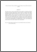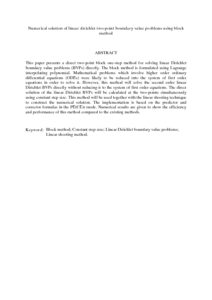# Numerical solution of linear dirichlet two-point boundary value problems using block method

## Citation

Hasni, Mohd Mughti and Abdul Majid, Zanariah and Senu, Norazak (2013) Numerical solution of linear dirichlet two-point boundary value problems using block method. International Journal of Pure and Applied Mathematics, 85 (3). pp. 495-506. ISSN 1311-8080; ESSN: 1314-3395

## Abstract

This paper presents a direct two-point block one-step method for solving linear Dirichlet boundary value problems (BVPs) directly. The block method is formulated using Lagrange interpolating polynomial. Mathematical problems which involve higher order ordinary differential equations (ODEs) were likely to be reduced into the system of first order equations in order to solve it. However, this method will solve the second order linear Dirichlet BVPs directly without reducing it to the system of first order equations. The direct solution of the linear Dirichlet BVPs will be calculated at the two-points simultaneously using constant step size. This method will be used together with the linear shooting technique to construct the numerical solution. The implementation is based on the predictor and corrector formulas in the PE(CE)r mode. Numerical results are given to show the efficiency and performance of this method compared to the existing methods.Preview
PDF (Abstract)
Numerical solution of linear dirichlet two.pdfView Item